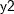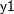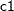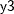The MI Procedure

MONOTONE Statement

• MONOTONE <method <(<imputed <= effects>> </ options>)>>
<…method <(<imputed <= effects>> </ options>)>>
;

The MONOTONE statement specifies imputation methods for data sets with monotone missingness. You must also specify a VAR statement, and the data set must have a monotone missing pattern with variables ordered in the VAR list.

Table 63.4 summarizes the options available for the MONOTONE statement.

Table 63.4: Summary of Imputation Methods in MONOTONE Statement

Option

Description

Specifies the discriminant function method

Specifies the logistic regression method

Specifies the propensity scores method

Specifies the regression method

Specifies the predictive mean matching method

For each method, you can specify the imputed variables and, optionally, a set of the effects to impute these variables. Each effect is a variable or a combination of variables preceding the imputed variable in the VAR statement. The syntax for specification of effects is the same as for the GLM procedure. See Chapter 45: The GLM Procedure, for more information.

One general form of an effect involving several variables is

X1 * X2 * A * B * C ( D E )

where `A`, `B`, `C`, `D`, and `E` are classification variables and `X1` and `X2` are continuous variables.

When a MONOTONE statement is used without specifying any methods, the regression method is used for all imputed continuous variables and the discriminant function method is used for all imputed classification variables. In this case, for each imputed continuous variable, all preceding variables in the VAR statement are used as the covariates, and for each imputed classification variable, all preceding continuous variables in the VAR statement are used as the covariates.

When a method for continuous variables is specified without imputed variables, the method is used for all continuous variables in the VAR statement that are not specified in other methods. Similarly, when a method for classification variables is specified without imputed variables, the method is used for all classification variables in the VAR statement that are not specified in other methods.

For each imputed variable that does not use the discriminant function method, if no covariates are specified, then all preceding variables in the VAR statement are used as the covariates. That is, each preceding continuous variable is used as a regressor effect, and each preceding classification variable is used as a main effect. For an imputed variable that uses the discriminant function method, if no covariates are specified, then all preceding variables in the VAR statement are used as the covariates with the CLASSEFFECTS=INCLUDE option, and all preceding continuous variables in the VAR statement are used as the covariates with the CLASSEFFECTS=EXCLUDE option (which is the default).

With a MONOTONE statement, the variables are imputed sequentially in the order given by the VAR statement. For a continuous variable, you can use a regression method, a regression predicted mean matching method, or a propensity score method to impute missing values. For a nominal classification variable, you can use either a discriminant function method or a logistic regression method (generalized logit model) to impute missing values without using the ordering of the class levels. For an ordinal classification variable, you can use a logistic regression method (cumulative logit model) to impute missing values by using the ordering of the class levels. For a binary classification variable, either a discriminant function method or a logistic regression method can be used.

Note that except for the regression method, all other methods impute values from the observed observation values. You can specify the following methods in a MONOTONE statement.

DISCRIM <( imputed < = effects> </ options> ) >

specifies the discriminant function method of classification variables. The available options are as follows:

CLASSEFFECTS=EXCLUDE | INCLUDE

specifies whether the CLASS variables are used as covariate effects. The CLASSEFFECTS=EXCLUDE option excludes the CLASS variables from covariate effects and the CLASSEFFECTS=INCLUDE option includes the CLASS variables as covariate effects. The default is CLASSEFFECTS=EXCLUDE.

DETAILS

displays the group means and pooled covariance matrix used in each imputation.

PCOV=FIXED | POSTERIOR

specifies the pooled covariance used in the discriminant method. The PCOV=FIXED option uses the observed-data pooled covariance matrix for each imputation and the PCOV=POSTERIOR option draws a pooled covariance matrix from its posterior distribution. The default is PCOV=POSTERIOR.

PRIOR=EQUAL | JEFFREYS <=c> | PROPORTIONAL | RIDGE <=d>

specifies the prior probabilities of group membership. The PRIOR=EQUAL option sets the prior probabilities equal for all groups; the PRIOR=JEFFREYS <=c> option specifies a noninformative prior, 0 < c < 1; the PRIOR=PROPORTIONAL option sets the prior probabilities proportion to the group sample sizes; and the PRIOR=RIDGE <=d> option specifies a ridge prior, d > 0. If the noninformative prior c is not specified, c=0.5 is used. If the ridge prior d is not specified, d=0.25 is used. The default is PRIOR=JEFFREYS.

See the section Monotone and FCS Discriminant Function Methods for a detailed description of the method.

LOGISTIC <( imputed < = effects> </ options> ) >

specifies the logistic regression method of classification variables.

The available options are as follows:

DESCENDING

reverses the sort order for the levels of the response variables.

DETAILS

displays the regression coefficients in the logistic regression model used in each imputation.

specifies the link function linking the response probabilities to the linear predictors. The default is LINK=LOGIT. The LINK=LOGIT option uses the log odds function to fit the binary logit model when there are two response categories and to fit the cumulative logit model when there are more than two response categories; and the LINK=GLOGIT option uses the generalized logit function to fit the generalized logit model where each nonreference category is contrasted with the last category.

ORDER=DATA | FORMATTED | FREQ | INTERNAL

specifies the sort order for the levels of the response variable. The ORDER=DATA sorts by the order of appearance in the input data set; the ORDER=FORMATTED sorts by their external formatted values; the ORDER=FREQ sorts by the descending frequency counts; and the ORDER=INTERNAL sorts by the unformatted values. The default is ORDER=FORMATTED.

See the section Monotone and FCS Logistic Regression Methods for a detailed description of the method.

PROPENSITY <( imputed < = effects> </ options> ) >

specifies the propensity scores method of variables. Each variable is either a classification variable or a continuous variable. The available options are DETAILS and NGROUPS=. The DETAILS option displays the regression coefficients in the logistic regression model for propensity scores. The NGROUPS= option specifies the number of groups created based on propensity scores. The default is NGROUPS=5.

See the section Monotone Propensity Score Method for a detailed description of the method.

REG | REGRESSION <( imputed < = effects> </ DETAILS> ) >

specifies the regression method of continuous variables. The DETAILS option displays the regression coefficients in the regression model used in each imputation.

With a regression method, the MAXIMUM=, MINIMUM=, and ROUND= options can be used to make the imputed values more consistent with the observed variable values.

See the section Monotone and FCS Regression Methods for a detailed description of the method.

REGPMM < ( imputed < = effects> < / options> ) >
REGPREDMEANMATCH < ( imputed < = effects > < / options > ) >

specifies the predictive mean matching method for continuous variables. This method is similar to the regression method except that it imputes a value randomly from a set of observed values whose predicted values are closest to the predicted value for the missing value from the simulated regression model (Heitjan and Little, 1991; Schenker and Taylor, 1996).

The available options are DETAILS and K=. The DETAILS option displays the regression coefficients in the regression model used in each imputation. The K= option specifies the number of closest observations to be used in the selection. The default is K=5.

See the section Monotone and FCS Predictive Mean Matching Methods for a detailed description of the method.

With a MONOTONE statement, the variables with missing values are imputed sequentially in the order specified in the VAR statement. For example, the following MI procedure statements use the default regression method for continuous variables to impute variablefrom the effect, the logistic regression method to impute variablefrom effects,, and, and the regression method to impute variablefrom effects,, and:

```proc mi;
class c1;
var y1 y2 c1 y3;
monotone logistic(c1= y1 y2 y1*y2);
monotone reg(y3= y1 y2 c1);
run;
```

The variableis not imputed since it is the leading variable in the VAR statement.